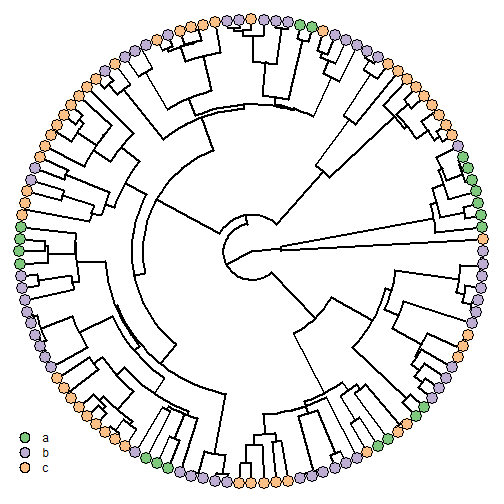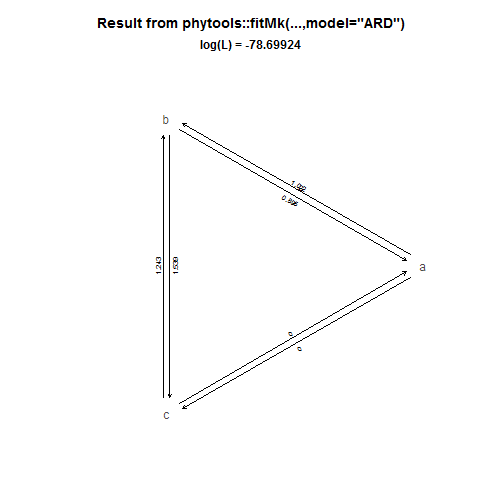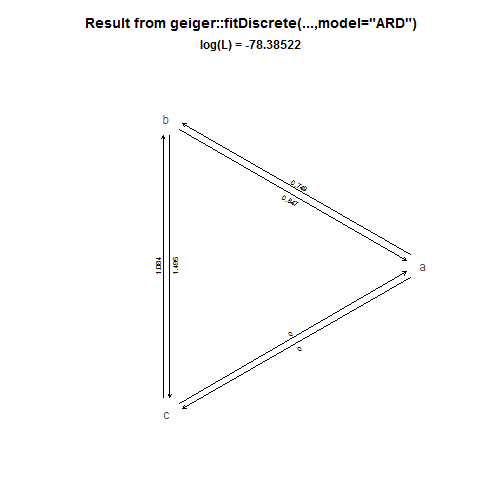## Wednesday, July 12, 2017

### Fitting discrete character (Mk) models with a fixed state at the root

“…how do I specify an ancestral state for my root node phytools?? And can I specify one when using the ER model of character evolution? I feel this should be really simple to answer, sorry but if you could direct me to an answer that would be fantastic.”

I'm going to interpret this as meaning “how do I fit a discrete character model in which the state at the global root is known.”

This is not super easy. It is most straightforward to do using `fitMk`, but can also be accomplished using `fitDiscrete` of the geiger package.

First I'll demonstrate `fitMk`.

Here are our tree & data:

``````library(phytools)
library(RColorBrewer)
states<-sort(unique(x))
plotTree(tree,type="fan",ftype="off")
tiplabels(tip=1:Ntip(tree),pie=to.matrix(x,states),
piecol=brewer.pal(3,"Accent"),cex=0.6)
states),shape="circle",prompt=FALSE,x=-1,y=-0.8)
``````Now, we're going to use an 'ordered' model - but in which we either use a flat prior probability distribution on the global root, or we fix the root to each of the three possible states:

``````model<-matrix(c(0,1,0,1,0,1,0,1,0),3,3,dimnames=list(states,
states))
model
``````
``````##   a b c
## a 0 1 0
## b 1 0 1
## c 0 1 0
``````
``````fit.flat<-fitMk(tree,x,model=model)
fit.flat
``````
``````## Object of class "fitMk".
##
## Fitted (or set) value of Q:
##           a         b         c
## a -1.204273  1.204273  0.000000
## b  1.204273 -2.408547  1.204273
## c  0.000000  1.204273 -1.204273
##
## Fitted (or set) value of pi:
##         a         b         c
## 0.3333333 0.3333333 0.3333333
##
## Log-likelihood: -79.285705
##
## Optimization method used was "nlminb"
``````
``````fit.a<-fitMk(tree,x,model=model,pi=setNames(c(1,0,0),states))
fit.a
``````
``````## Object of class "fitMk".
##
## Fitted (or set) value of Q:
##           a         b         c
## a -1.266891  1.266891  0.000000
## b  1.266891 -2.533781  1.266891
## c  0.000000  1.266891 -1.266891
##
## Fitted (or set) value of pi:
## a b c
## 1 0 0
##
## Log-likelihood: -80.385615
##
## Optimization method used was "nlminb"
``````
``````fit.b<-fitMk(tree,x,model=model,pi=setNames(c(0,1,0),states))
fit.b
``````
``````## Object of class "fitMk".
##
## Fitted (or set) value of Q:
##           a         b         c
## a -1.148579  1.148579  0.000000
## b  1.148579 -2.297158  1.148579
## c  0.000000  1.148579 -1.148579
##
## Fitted (or set) value of pi:
## a b c
## 0 1 0
##
## Log-likelihood: -78.905018
##
## Optimization method used was "nlminb"
``````
``````fit.c<-fitMk(tree,x,model=model,pi=setNames(c(0,0,1),states))
fit.c
``````
``````## Object of class "fitMk".
##
## Fitted (or set) value of Q:
##           a         b         c
## a -1.251647  1.251647  0.000000
## b  1.251647 -2.503294  1.251647
## c  0.000000  1.251647 -1.251647
##
## Fitted (or set) value of pi:
## a b c
## 0 0 1
##
## Log-likelihood: -79.059712
##
## Optimization method used was "nlminb"
``````

Note that although one might be tempted to compare the likelihoods of these three fitted models, this should not in fact be done. This is because the likelihoods are in each case conditioned on the state at the root state being known - in other words, they are conditioned on different data. That this is not equivalent to constraining a particular parameter to have some value is most evident by comparison between the model in which the root is fixed to state `"b"` to the model in which the root state is unknown. If the former was a special case of the latter, then it should have a lower (or equal) likelihood, when in fact its likelihood is slightly higher. How come? Well in reality the fixed state likelihood is the probability that all the tips are in their observed states and that the root is in state `"b"`, given the tree, a model, and the model parameters (to be estimated); whereas the 'flat prior' model likelihood is simply the probability of the data at the tips, given the tree & model, but integrating over all possible values for the root node of the phylogeny.

We can do the same analysis using `fitDiscrete`, but it is not quite as straightforward. First, we have to use the method to generate a likelihood function, and then we can optimize that function using different prior probability distributions for the root of the tree, as follows:

``````library(geiger)
obj<-fitDiscrete(tree,x,model=model)
``````
``````## Warning in fitDiscrete(tree, x, model = model): Parameter estimates appear at bounds:
##  q13
##  q31
``````
``````obj
``````
``````## GEIGER-fitted comparative model of discrete data
##  fitted Q matrix:
##               a         b         c
##     a -1.184179  1.184179  0.000000
##     b  1.184179 -2.368358  1.184179
##     c  0.000000  1.184179 -1.184179
##
##  model summary:
##  log-likelihood = -79.073420
##  AIC = 160.146839
##  AICc = 160.180738
##  free parameters = 1
##
## Convergence diagnostics:
##  optimization iterations = 100
##  failed iterations = 0
##  frequency of best fit = 1.00
##
##  object summary:
##  'lik' -- likelihood function
##  'bnd' -- bounds for likelihood search
##  'res' -- optimization iteration summary
##  'opt' -- maximum likelihood parameter estimates
``````

(Note that `fitDiscrete` does not use a flat prior by default.)

``````lik<-obj\$lik
lik
``````
``````## likelihood function for univariate discrete trait evolution
##  argument names: q12
##
##  mapping
##      1: a
##      2: b
##      3: c
##
## definition:
## function (pars, ...)
## {
##     if (missing(pars))
##         stop(paste("The following 'pars' are expected:\n\t",
##             paste(argn(tmp), collapse = "\n\t", sep = ""), sep = ""))
##     pars = .repars(pars, argn(tmp))
##     tmp(pars, ...)
## }
## <bytecode: 0x00000000086ba470>
## <environment: 0x000000000bcfb828>
``````
``````fit.flat<-optimize(lik,c(1e-8,1000),root="flat",maximum=TRUE)
fit.flat
``````
``````## \$maximum
##  1.20427
##
## \$objective
##  -79.2857
``````
``````fit.a<-optimize(lik,c(1e-8,1000),root="given",
root.p=setNames(c(1,0,0),states),maximum=TRUE)
fit.a
``````
``````## \$maximum
##  1.266875
##
## \$objective
##  -80.38561
``````
``````fit.b<-optimize(lik,c(1e-8,1000),root="given",
root.p=setNames(c(0,1,0),states),maximum=TRUE)
fit.b
``````
``````## \$maximum
##  1.148578
##
## \$objective
##  -78.90502
``````
``````fit.c<-optimize(lik,c(1e-8,1000),root="given",
root.p=setNames(c(0,0,1),states),maximum=TRUE)
fit.c
``````
``````## \$maximum
##  1.251644
##
## \$objective
##  -79.05971
``````

For each fitted model, `\$maximum` is the single fitted model parameter; whereas `\$objective` is the log-likelihood. If we had a model with more than one parameter, we could use `optim` or some other numerical optimizer to fit the model.

Finally, don't forget that phytools has S3 plotting methods for both `fitMk` & `fitDiscrete`. E.g.:

``````plot(fit.phytools<-fitMk(tree,x,model="ARD"))
title(main="Result from phytools::fitMk(...,model=\"ARD\")")
title(main=paste("\n\n\nlog(L) =",round(logLik(fit.phytools),5)),
cex.main=1)
````````````plot(fit.geiger<-fitDiscrete(tree,x,model="ARD"))
title(main="Result from geiger::fitDiscrete(...,model=\"ARD\")")
title(main=paste("\n\n\nlog(L) =",round(logLik(fit.geiger),5)),
cex.main=1)
``````(Note that these two fitted models, and their likelihoods, come out as different - but in reality the likelihoods are not directly comparable because the two functions make different assumptions about the prior on the root. If we force geiger's `fitDiscrete` likelihood function to use a flat prior, we should find that it yields a worse likelihood then we obtained using `phytools:::fitMk` - if, in fact, `fitMk` has converged on the MLE, e.g.:

``````lik<-fit.geiger\$lik
q<-fit.phytools\$rates
## fitMk paramater estimates
lik(pars=c(q12=q,q13=q,q21=q,q23=q,q31=q,q32=q),
root="flat")
``````
``````##  -78.69924
``````
``````## fitDiscrete estimates, but with flat root prior
lik(pars=unlist(fit.geiger\$opt[1:6]),root="flat")
``````
``````##  -78.77036
``````

It would also have been plausible that `fitMk` had failed to converge on the ML solution as, in general, the optimization routines used by `fitDiscrete` are more robust. This doesn't seem to the case in this instance.)

The data for this exercise were simulated as follows:

``````tree<-pbtree(n=120,scale=1)
Q<-matrix(c(-1,1,0,1,-2,1,0,1,-1),3,3,dimnames=list(letters[1:3],
letters[1:3]))
x<-as.factor(sim.history(tree,Q,anc="b")\$states)
``````

That's it.

#### 1 comment:

1.Dear Liam, many thanks for this very enlightening post!
While trying to perform some reconstructions, I came accross an unexpected result. Iam trying to test different scenarios for character evolution (equal rates, all-rates-different, and customized matrices with irreversible states).

Specifically, I have a character that has a defined ancestral condition (established by outgroups not present in the analysis), three different derived conditions, and taxa which lack this particular structure. A small group of taxa which is deeply nested in the tree presents the plesiomorphic condition, and I would like to test if this is a plesiomorphic retention or a re-gain. In the all-rates-different model, it is optimized as a re-gain. So I wanted to compare this with a model where re-gains are impossible, using this matrix:
- "ancestral" can shift to any state
-"derived" states can shift to "absent" only, but not to other derived states, nor reverse to the "ancestral" condition
-"absent" cannot shift to any condition

This optimizes the character as ancestral. Everything seems fine so far!

However, the exact same tree is produced if a use a different matrix where all transitions are possible except for re-gains from "absent" structures (i.e.the model allows for reversals from the derived states to the ancestral state, and also transitions among derived states). I find this very strange, because the only difference between this and the ARD model is the impossibility to reverse from "absent" to other states - but neither of the trees suggested such an optimization would occur. Thus, I would expect that this second customized matrix would give results identical to those of ARD -- do you know why such a result should to be expected?

Note: due to the very large amount of spam, all comments are now automatically submitted for moderation.